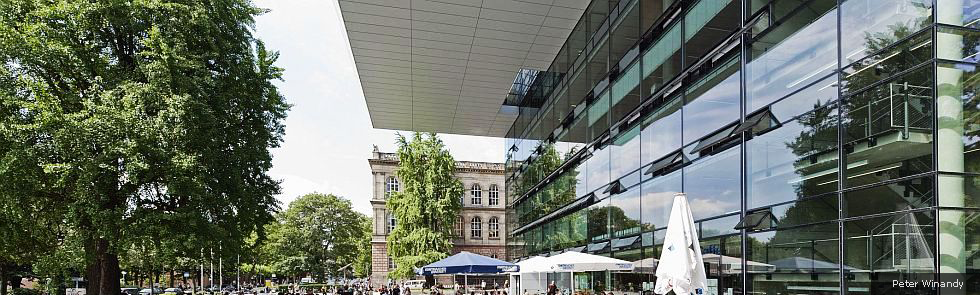Kategorien
Seiten
-• Diese Veranstaltung hat bereits stattgefunden.

# EU Regional School with Prof. Dr. Raul F. Tempone

## Prof. Dr. Raul F. Tempone – Forward and Inverse Problems with Multilevel Monte Carlo

### Abstract

We will first recall, for a general audience, the use of Monte Carlo and Multi-level Monte Carlo methods in the context of Uncertainty Quantification. Then we will discuss our Adaptive Multilevel Monte Carlo (MLMC) Methods for (i) Itô Stochastic Differential Equations, (ii) Stochastic Reaction Networks modeled by Pure Jump Markov Processes and (iii) Partial Differential Equations with random inputs. In this context, the notion of adaptivity includes several aspects such as mesh refinements based on either a priori or a posteriori error estimates, the local choice of different time-stepping methods, pathwise dependent importance sampling, and the selection of the total number of levels and the number of samples at different levels. Our Adaptive MLMC estimator uses a hierarchy of adaptively refined, non-uniform time discretizations. Thus, it is a generalization of the uniform discretization MLMC method introduced independently by M. Giles and S. Heinrich. Our adaptive MLMC algorithms are provably asymptotically accurate and have the correct complexity with improved characterization of the multiplicative constant factor in the asymptotic analysis. In this context, we developed the Continuation Multilevel Monte Carlo (CMLMC) for the estimation of parameters needed in our MLMC algorithms, such as the variance of the difference between consecutive approximations. These techniques take particular care of the deepest levels, where for efficiency reasons, only a few realizations are available to produce essential estimates. Moreover, we show the asymptotic normality of the statistical error in the MLMC estimator, justifying in this way, our error estimate that allows prescribing both the required accuracy and confidence level in the final result.
We will also briefly mention some recently smoothing techniques for rough observables and the approximation of probability density functions.
We will comment on their use for Ito diffusions and the non-Markovian rough Bergomi model. This recent model has very low pathwise regularity, making the direct application of multilevel Monte Carlo inefficient.
Finally, we will comment on our multilevel works on inverse problems, data assimilation, optimal experimental design, and regression, hinting on the challenges addressed and the gains obtained so far.
We present several examples to illustrate the above results and the corresponding computational savings.
References:
1. „A Continuation Multilevel Monte Carlo algorithm“, by N. Collier, A.-L. Haji-Ali, F. Nobile, E. von Schwerin and R. Tempone. BIT Numer. Math., 55, pp. 399–432, 2015.
2. „Optimization of mesh hierarchies in Multilevel Monte Carlo samplers of SDEs and PDEs with stochastic coefficients“, by A.-L. Haji-Ali, F. Nobile, E. von Schwerin and R. Tempone. Stochastic Partial Differential Equations: Analysis and Computations, Vol. 4, Issue 1, Pages 76–112, 2016.
3. „Implementation and Analysis of an Adaptive Multi-Level Monte Carlo Algorithm“, by H. Hoel, E. von Schwerin, A. Szepessy, and R. Tempone. Monte Carlo Methods and Applications, 20(1), pp. 1–41, 2014.
4. „Construction of a mean square error adaptive Euler-Maruyama method with Applications in Multilevel Monte Carlo“, by H. Hoel, J. Happola and R. Tempone. Springer Proceedings in Mathematics & Statistics, Vol. 163 (2016), Monte Carlo and Quasi-Monte Carlo Methods, MCQMC, Leuven, Belgium, April 2014.
5. „Multilevel Hybrid Chernoff Tau-leap,“ by A. Moraes, R. Tempone, and P. Vilanova. BIT Numerical Mathematics, Volume 56, Issue 1, pp 189-239, 2016.
6. „A multilevel adaptive reaction-splitting simulation method for stochastic reaction networks“ by A. Moraes, R. Tempone, and P. Vilanova. SIAM Journal on Scientific Computing (SISC), 38 (2016), no. 4, A2091–A2117.
7. „Multilevel drift-implicit tau-leap“, by C. Ben Hammouda, A. Moraes and R. Tempone. Journal of Numerical Algorithms 74, no. 2, 527–560, 2017.
8. „Importance sampling for a robust and efficient multilevel Monte Carlo estimator for stochastic reaction networks“, by C. Ben Hammouda, N. Ben Rached and R. Tempone. arXiv:1911.06286, November 2019. To appear in Statistics and Computing.
9.“Multilevel Double Loop Monte Carlo and Stochastic Collocation Methods with Importance Sampling for Bayesian Optimal Experimental Design“, by
J. Beck, B.M. Dia, L. Espath, and R. Tempone. November 2018.
To appear in International Journal for Numerical Methods in Engineering, 2020.
10. „Multilevel weighted least-squares polynomial approximation“, by A.-L. Haji-Ali, F. Nobile, R. Tempone, and S. Wolfers. „Multilevel weighted least squares polynomial approximation“, by A.-L. Haji-Ali, F. Nobile, R. Tempone and S. Wolfers. ESAIM: Mathematical Modelling and Numerical Analysis (M2AN) 54 (2020) 649–677.
11. „Multilevel ensemble Kalman filtering“, by H. Hoel, K. J. H. Law and R. Tempone, SIAM Journal of Numerical Analysis, (SINUM), 54 (2016), no. 3, 1813–1839.
12. ”Multilevel ensemble Kalman filtering for spatially extended models”, by A. Chernov, H. Hoel, K. Law, F. Nobile and R. Tempone. arXiv:1608.08558v1, August 2016.

Datum:
17. Juli 2020
Zeit:
9:00 - 12:00

IRTG-2379 MIP

## Veranstaltungsort

a link for the Zoom meeting room will be send in the newsletter one week before the seminar starts. If you need any organizational help please contact office@aices.rwth-aachen.de